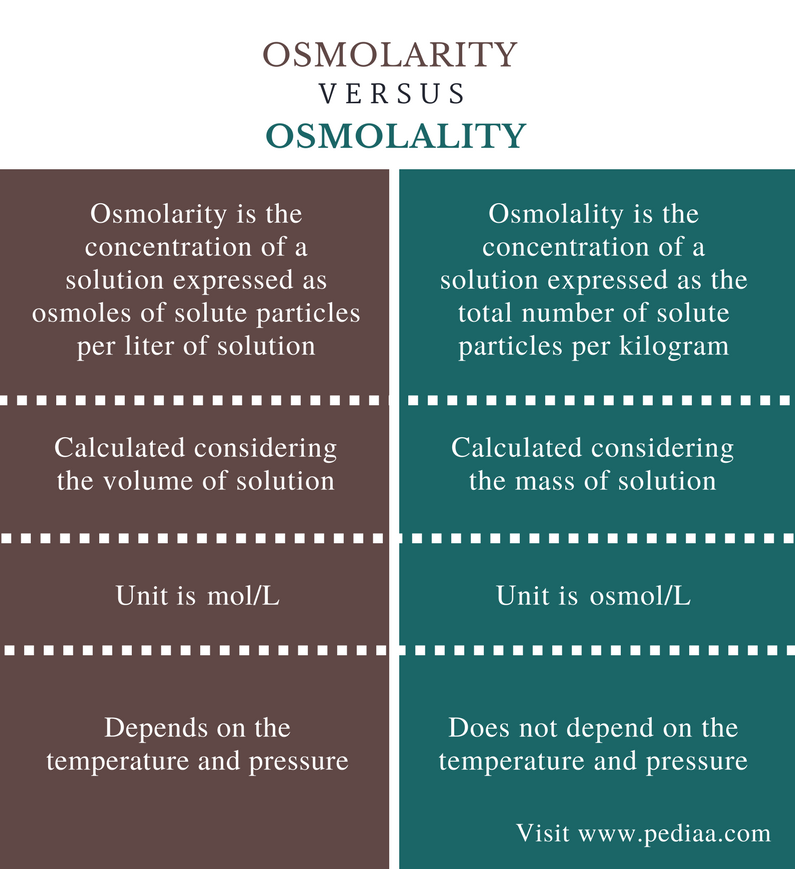# Osmolarity | Definition, Formula & Osmolarity vs. Osmolality

### Osmolarity

Osmolarity is basically the degree of how many solute osmoles are existing in 1 liter of a standard solution. These are consequential values are derived from the Na+, K+ ions, Glucose and Urea concentrations. Their values are variable in numerous conditions. Similarly, in other words, it is defined as an approximation of the Osmole concentration of the Plasma and it is proportionate to the element numbers per Liter of the standard solution. It is expressed as mmol/L. When a premeditated value is derived when it is used.

These two following equations can be utilized :

Osmolarity = 2 (Na+) + 2 (K+) + Glucose + Urea (all in mmol/L)

Osmolarity = 2 (Na+) + Glucose + Urea (all in mmol/L).

### The Formula of Osmolarity:�

Osmolarity= (number of moles of dissolved particles) / (numbers of liters of solution)

### How to Calculate Osmolarity from Molarity?

To calculate Osmolarity multiply the Molarity by the number of Osmoles that each solute produces.

#### Explanation:

An osmole (Osmol) is basically 1 mol particles that contribute to generating Osmotic Pressure of a solution.

For example, when NaCl dissolves in water it completely dissociates in water and forms Na+�ions and�Cl�ions.

Thus, each one mole of NaCl becomes two osmoles in solution after dissociation: one mole of Na+ ion and one mole of Cl– ion.

A solution of 1 mol/L�NaCl�has an�osmolarity�of 2 Osmol/L.

Also, a solution of 1 mol/L�CaCl2�has an osmolarity of 3 Osmol/L (1 mol�Ca2+�and 2 mol�Cl).

##### Example:### What Is an Osmole?

Osmole is not recognized as the SI unit of measurement it is also not considered the part of SI unit which means that it hasn’t been consistent. It is basically the measurement of the no. of moles of a solute that subsidize to create the Osmotic pressure of any chemical or biochemical solution.

Besides this Osmole has not been considered the standardized unit when Solution�s Osmotic Pressure is inconsequential, so another unit may be used to measure the no. off moles of solute inside the solution. And the unit is millimoles per liter.

### Osmotic Concentration

In simple words solution�s osmolarity is an amount of how much solute is strenuous or concentrated inside the solution of 1 liter. That is measured in osmoles (Osm) unit. Whereas osmotic concentration in actuality written as osmoles per liter (Osm/L).

In some occasions, osmotic concentration is measured in millimoles per liter (mmol/L). If in the solution amount of solvent or water increased the osmotic concentration of the solute declined. But if in the solution amount of solvent or water decreased the osmotic concentration of the solute amplified.

### What Is Osmotic Pressure?

Term Osmolarity is meticulously tangled with the concept of Osmosis, due to this Osmole is utilized to measure the solution�s osmotic concentration.

In simple words solution�s Osmotic Pressure is defined as how much amount of pressure is obligatory to generate an Equilibrium within the solution by moving a solution by a Semipermeable membrane. In other word solution�s osmolarity can also be stated that how much osmoles concentration is obligatory or needed to generate the Equilibrium.

### Osmolality

Osmolality is defined as Plasma�s Osmolar concentration. Osmolality is proportionate to the particles numbers per kilogram (kg) of the solvent. Osmolality is articulated or represented as mOsmol/kg. its SI unit is mmol/kg but then mOsmol/kg is also tranquil extensively used.

In the clinical laboratories, Osmolality is measured by using a special instrument Osmometer. The standard osmolality of extracellular fluid is 280-295 mOsmol/kg.

### Osmolarity vs. Osmolality

Sometime Osmolarity is taken alongside with term osmolality. Both are interrelated to the measurement of osmoles’ concentration within a solution. The principal difference between these two terms is that Osmolarity is the measurement of osmoles numbers within a liter of a solution, while Osmolarity measures the number of osmoles per kilogram (Osm/kg) of solvent. Both are written in terms of millimoles per kilogram (mmol/kg) in some instances.